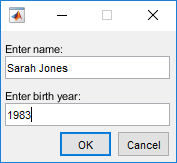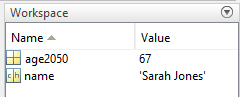# assignin

Assign value to variable in specified workspace

## Syntax

``assignin(ws,var,val)``

## Description

example

``assignin(ws,var,val)` assigns the value `val` to the variable `var` in the workspace `ws`. For example, `assignin('base','x',42)` assigns the value 42 to the variable `x` in the MATLAB® base workspace.If `val` requires evaluation, MATLAB evaluates it in the function that calls `assignin`, not in the workspace specified by `ws`. If `val` is a function handle, it must be evaluable in the function that calls `assignin`.The `assignin` function is useful for these tasks:Exporting data from a function to the base workspace.From within a function, changing the value of a variable that is defined in the workspace of the caller function. For example, you can change the value of a variable in the calling-function argument list.`

## Examples

collapse all

In a file in your current working folder, create a function that adds two numbers and then assigns a value to a variable `fcnStatus` in the base workspace.

```function c = myAdd(a,b) c = a+b; str = sprintf('%s called with %d,%d (%s)',mfilename,a,b,char(datetime)); assignin('base','fcnStatus',str) end```

At the command prompt, call the function.

`n = myAdd(2,3)`
```n = 5```

View the value of the `fcnStatus` variable that the `myAdd` function assigned in the base workspace.

`fcnStatus`
```fcnStatus = 'myAdd called with 2,3 (17-Nov-2017 14:56:14)'```

In a file in your current working folder, create a function that displays a dialog box to input a name and a birth year and computes age in the year 2050. The `assignin` function exports the values to the MATLAB workspace variables `name` and `age2050`.

```function mydialog prompt = {'Enter name:','Enter birth year:'}; answer = inputdlg(prompt); n = answer{1}; birthyear = str2double(answer{2}); a = 2050-birthyear; assignin('base','name',n); assignin('base','age2050',a); end```

At the command prompt, run the function, enter data, and click OK.

`mydialog`View the exported values in the Workspace browser.Create a function that changes an input age to 42. The call to `assignin` in `localfcn` changes the value of `a` in the workspace of the main function, `updateAge`.

```function updateAge(a) validateattributes(a,{'numeric'},{'scalar'}) fprintf('\tYour age: %d\n',a) localfcn fprintf('\tYour updated age: %d\n',a) end function localfcn assignin('caller','a',42) end```

At the command prompt, call the main function.

`updateAge(37)`
``` Your age: 37 Your updated age: 42```

While this example describes how to assign a variable into the caller workspace, the best practice is to have the local function `localfcn` return the updated age as an output argument.

In a file in your current working folder, create a function that finds the minimum value of a random array. The `assignfh` local function assigns the function handle `fh` into the workspace of `minRand`. The `minRand` function evaluates `fh` with the input `n`.

```function m = minRand(n) assignfh A = fh(n) m = min(A(:)); end function assignfh fh = @(dim)rand(dim); assignin('caller','fh',fh) end```

Call the function with an input value of 2.

`m = minRand(2)`
```A = 0.3486 0.1423 0.0419 0.0766 m = 0.0419```

The function handle evaluates to a 2-by-2 array of random numbers.

Create another version of the function, called `minRand2`, in which the local function overrides the `rand` function in the function handle definition.

Similar to the `minRand` example, the `assignfh2` local function assigns `fh` into the workspace of `minRand2`. The `assignfh2` function overrides the `rand` function in its workspace with a variable named `rand` and creates the function handle. This behavior is consistent with anonymous functions – the function handle is created using variables available at the time you create it. Therefore, the function handle evaluation in `minRand2` results in `n` indexing into the `rand` array defined in `assignfh2`.

```function m = minRand2(n) assignfh2(n) A = fh(n) m = min(A(:)); end function assignfh2(n) rand = 13*ones(n); fh = @(dim)rand(dim); assignin('caller','fh',fh) end```

Call the function with an input value of 2.

`m = minRand2(2)`
```A = 13 m = 13```

When assigning an anonymous function to a caller workspace, MATLAB puts the definition of the function handle in a variable in the caller workspace. The function with the call to `assignin` evaluates the function handle. While this example describes how to assign a variable into the caller workspace, the best practice is to have the local function `assignfh` return the function handle as an output argument.

## Input Arguments

collapse all

Workspace, specified as `'base'` or `'caller'`.

To assign values in the MATLAB base workspace, use `'base'`. The base workspace stores variables that you create at the MATLAB command prompt, including any variables that scripts create, assuming that you run the script from the command line or from the Editor.

To assign variables in the workspace of the caller function, use `'caller'`. The caller workspace is the workspace of the function that called the currently running function. For example, assume that `funA` calls `funB`. The caller workspace of `funB` is `funA`. Therefore, from `funB`, you can assign a value to a variable in `funA` using `assignin` and specifying the workspace as `'caller'`.

Note

Assigning to variables in the caller workspace can make code more difficult to understand, give surprising results to the user (unexpected or redefined variables in their workspace), and have a negative performance impact. The best practice is to have the function return the variables as output arguments.

The base and caller workspaces are equivalent in the following cases:

• You call a function at the command prompt and the main function calls `assignin`.

• You call `assignin` at the command prompt.

Data Types: `char` | `string`

Variable name, specified as a character vector or string scalar. If `var` does not exist in the specified workspace, the `assignin` function creates it.

Data Types: `char` | `string`

Value of variable, specified as a scalar or array value. `val` can have any data type, and can include MATLAB expressions.

If the value of the variable requires evaluation, MATLAB evaluates the expression in the function that contains the call to `assignin`, not in the workspace specified by `ws`. If `val` is a function handle, it must be evaluable in the function that calls `assignin`.

Example: `5`

Example: `'hello'`

Example: `rand(3,7)`

Example: `@cos`

## Tips

• The `assignin` function does not assign values to specific elements of an array. Therefore `var` cannot contain array indices. This code results in an error.

```X = 1:8; assignin('base','X(3:5)',-1); ```

To assign values to specific elements of an array, use the `evalin` function.

```evalin('base','X(3:5) = -1') ```

## Version History

Introduced before R2006a